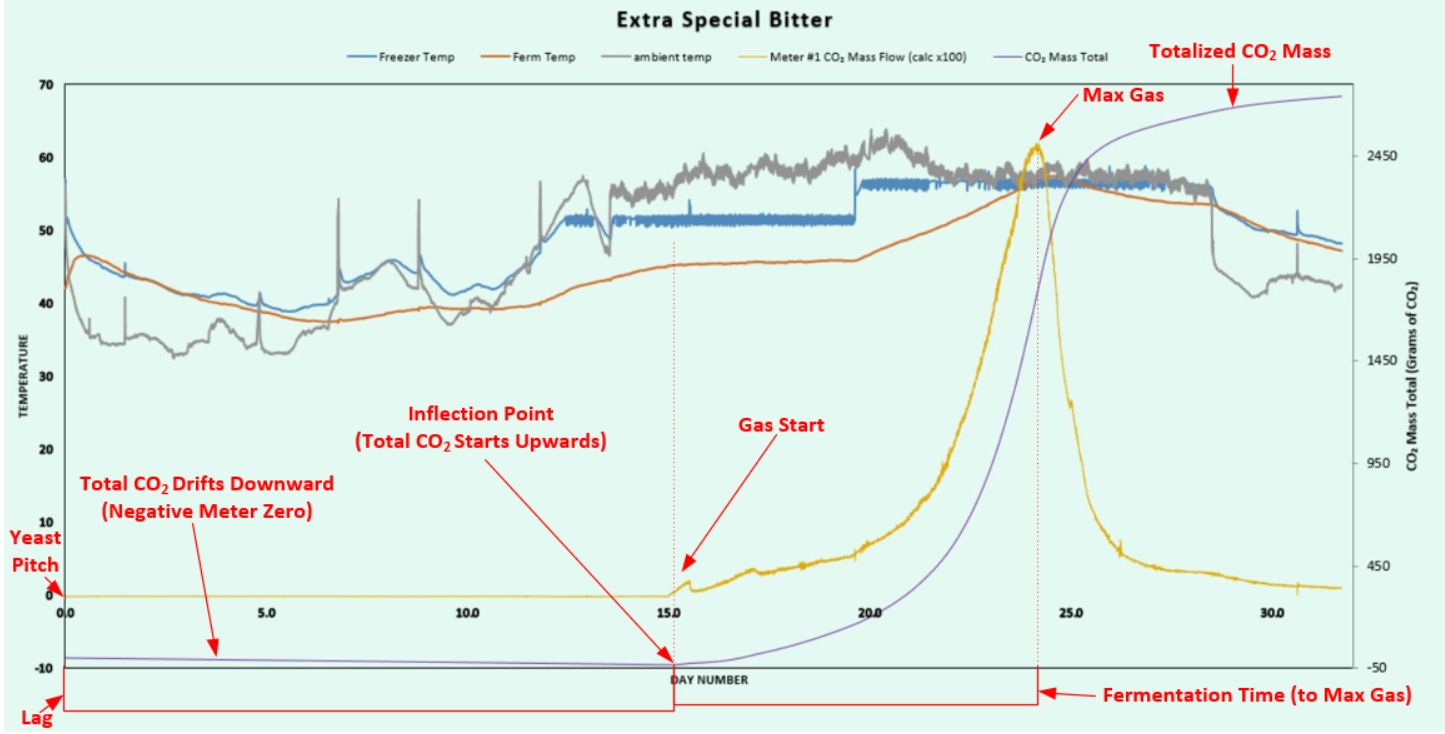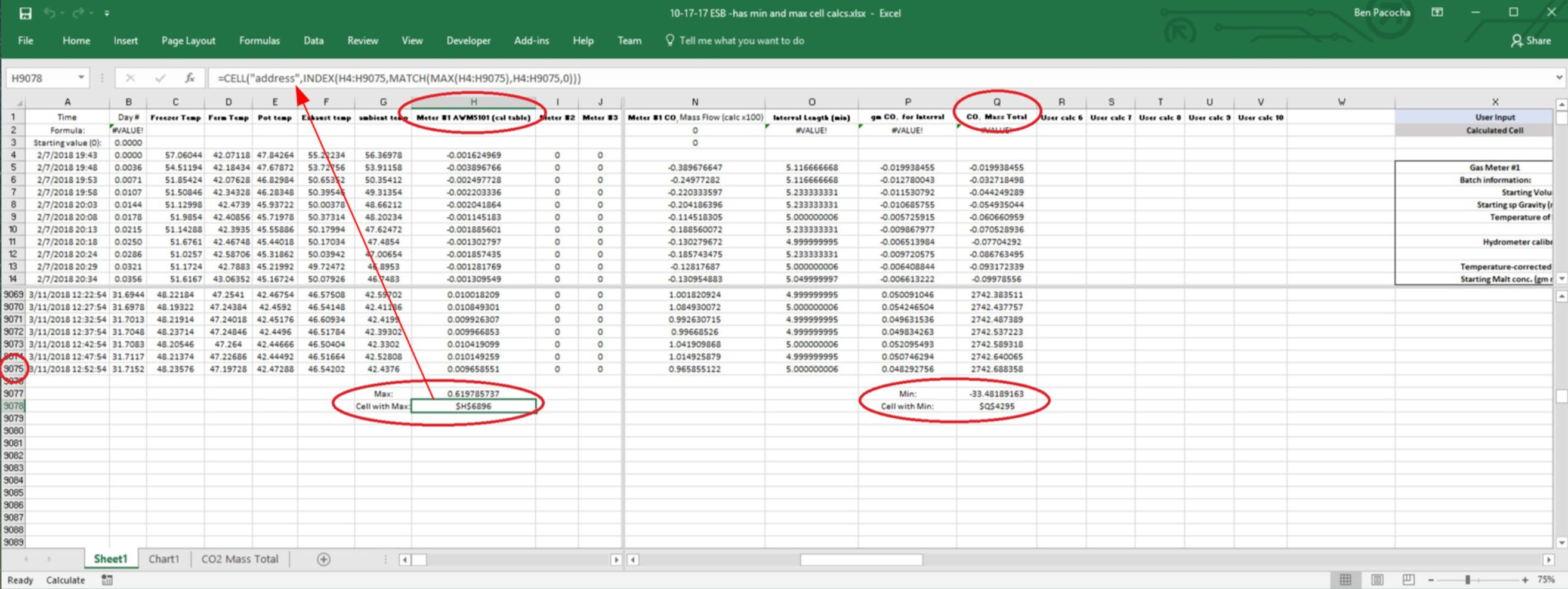# Batch Score - Excel™ Cheat Sheet

## Acquiring Batch Score Values From Excel™ Batch Data

You are looking for two values from the batch spreadsheet:

• Gas Start
• Max Gas

## Gas Start

Firstly, with ale fermentations, the gas start is so clear and distinct in the data, that no further mathematical process is needed. Just find the gas start peak on the Excel™ graph and let that send you to the correct row to copy and paste the gas start info into the Batch Score sheet.Negative Meter-Zero Example - Purple Line is CO2 Mass Total

With lagers the bubbles may start slow. This lack of clarity makes us choose a rigorous way to assign the "Gas Start" timing. The batches will all be compared to each other, so these values must be unambigously determined. For this you need to know if the meter zero was positive or negative. This is seen as a negative or positive value for the beginning values in the Meter #1 (cal table) raw data in column H. Once you run through this a couple of times, you will find that this is not a big deal.

Easiest is negative! If the first Meter #1 values (cell H4 and on) are negative, then the CO2 Mass Total (probably column Q) is going to build in a negative direction until the bubbles start. At this point the gas total will start building in a positive direction until the end. So we are looking for most negative gas mass total value in column Q. After this, the bubbles will drive the remaining entries higher.

You need to use Excel™ to find the most negative value in column Q, and the day number this occured on. First, split the screen on the worksheet to find the last row of data. This row number could be anything depending on how long the batch data capture was run. At the very bottom of column Q, you want it to look like this:

Min: -0.321752154
Cell with Min: \$Q\$48Batch Data With Min and Max Calculations

The Excel™ formula for Min is:

=MIN(Q5:Q1275)

...with Q1275 being replaced with the last cell of data in your sheet. Let's say our new batch sheet has data until Q9075:

=MIN(Q5:Q9075)

The Excel™ formula for the cell address that contains the Min is:

...replace any occurrence of 1275 with 9075 (3 spots), and place that formula in the sheet:

You now know what the minimum was in column Q, and where to find it. Place the Minimum value in the Batch Score spread sheet. Get to the row specified for the Min, and also place the Day number for that row in the spread sheet (copy the number from the batch sheet and paste "values" into the Batch Score sheet.

For a positive meter zero (beginning Meter #1 values (cell H4 and on) are positive):

This is a little harder, because there is no clear inflection point in the gas mass total from negative to positive values. The mass total increments slowly when there are no bubbles, then quickens when the fermentation starts. The best we can do in this case is to use a fraction of the ending mass total to derive the "Gas Start" timing. I am getting good results using 0.25% of the ending mass total. This places the gas start where you can see things start to happen on the graph. You can choose a different value, but you have to use the same value for all of your batches that you are comparing.

The process is:

multiply the ending mass total by 0.0025 (0.25%). This gives you a starting mass total to search for in Column Q. Find the two rows with values lower and higher then the calculated one. The row with the lower one is your "Gas Start" row.

In the Amber IPA data, my last row of data is row 6131. The Mass Total value (for Meter #1) in cell Q6131 is: 3082.690214

3082.690214 x .0025 = 7.71 grams CO2

Looking for the 7.71 value in the rows of column Q, I find that it is reached between rows 348 & 349. So, I will use row 348 as the gas start row for the Batch Score sheet. You can comfirm this day number value makes sense by looking at the graph.

## Max Gas

The maximum gas is the peak gas flow achieved in the raw meter data (Column H for Meter #1). All you have to do here is run the Excel™ formula to find the max value in column H, navigate to that row, and document this rate and its corresponding Day Number value in the Batch Score sheet.

You want the bottom of the data in column H to look like this:

Max: 0.371920074
Cell with Max: \$H\$3384

Max formula:

=MAX(H4:H6131)

Address of Cell with Max formula:

In this case, my Max rate is found in cell H3384. Once I navigate to that row, I need to also record the day number and fermentation temperature into the Batch Score sheet. The ferm temperature is in column D on the Amber IPA example sheet.

Notes:

For the batches to compare to each other, the run settings have to match. For example, a 1-minute row interval will give a higher peak flow rate then a 5-minute row interval. These two cannot be compared in the same Batch Score sheet.

## Excel™ Formulas for Max Gas calculation:

Max Gas (maximum individual meter reading; column H for meter 1):

=MAX(H4:H6131)